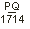Call 1-800-728-5168 | Email Us# Basic Hydraulic Formula Table

Get back to the basics with these basic hydraulic pump calculations – fluid pressure, fluid flow rate, and fluid power. Need more information about hydraulic pumps? Check out our other hydraulic formulas and resources.  Need a hydraulic pump repair service? Contact us today.

## Hydraulic Formula Table

Formula For: Word Formula Letter Formula
Fluid Pressure
In Pounds/Square Inch
Pressure =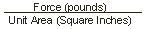P =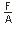or
psi =Fluid Flow Rate
In Gallons/Minute
Flow Rate =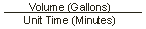Q =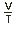Fluid Power
In Horsepower
Horsepower =HP =